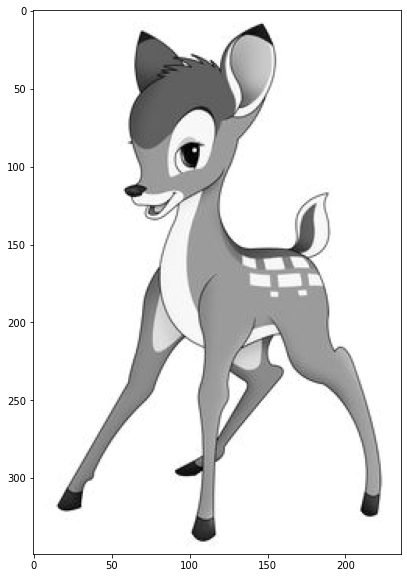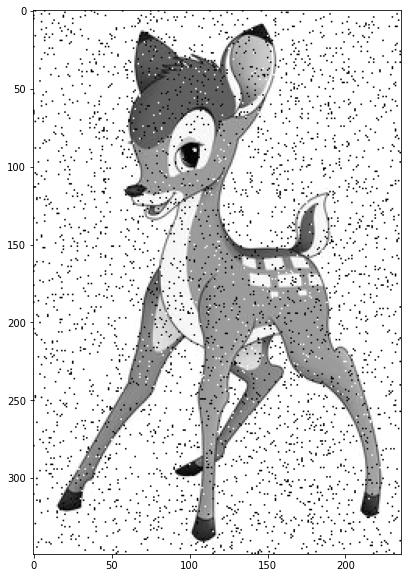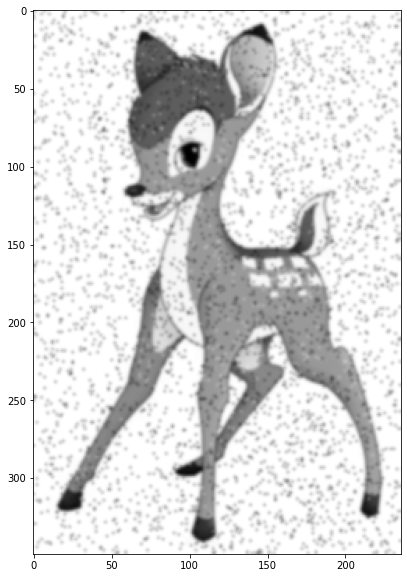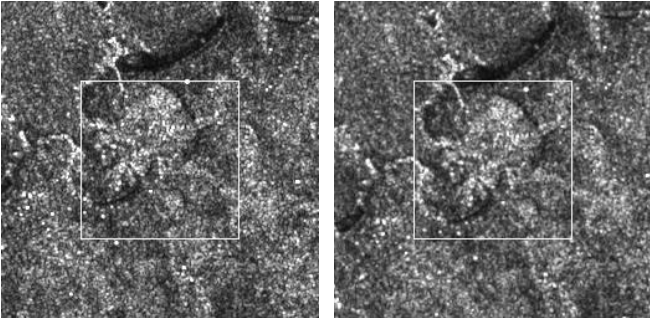# Median Filtering¶

Faisal Qureshi
Professor
Faculty of Science
Ontario Tech University
http://vclab.science.ontariotechu.ca

## Lesson Plan¶

• Median filtering
In :
import cv2 as cv
import numpy as np
import matplotlib.pyplot as plt


## Salt-and-pepper noise¶

Lets add some salt-and-pepper (or impulse) noise to the image. This noise can be caused by sharp and sudden disturbances in the image signal. It presents itself as sparsely occurring white and black pixels.

In :
im = cv.imread('data/bambi.jpg',0).astype(np.uint8)

plt.figure(figsize=(10,10))
plt.imshow(im, cmap='gray')

Out:
<matplotlib.image.AxesImage at 0x1252fc410>Lets add some salt and pepper noise

In :
black = 10
white = 250

plt.figure(figsize=(10,10))
plt.imshow(im, cmap='gray')

Out:
<matplotlib.image.AxesImage at 0x125aea410>## Gaussian smoothing to get rid of salt-and-pepper noise¶

The following example supports the assertion that Gaussian smoothing fails to get rid of the salt-and-pepper noise.

In :
half_width = 2
sigma = 1

plt.figure(figsize=(10,10))
plt.imshow(cv.GaussianBlur(im, (2*half_width+1, 2*half_width+1), sigma), cmap='gray')

Out:
<matplotlib.image.AxesImage at 0x125ce6110>## Median filtering¶

This is a non-linear filtering technique. (What does that mean?) It is widely used in digital image processing and signal processing to get rid of speckle and salt-and-pepper noise while preserving edges.

From Wikipedia article on median filtering

For small to moderate levels of Gaussian noise, the median filter is demonstrably better than Gaussian blur at removing noise whilst preserving edges for a given, fixed window size. However, its performance is not that much better than Gaussian blur for high levels of noise, whereas, for speckle noise and salt-and-pepper noise (impulsive noise), it is particularly effective. Because of this, median filtering is very widely used in digital image processing.

Aside: Speckle is a granular interference that inherently exists in and degrades the quality of the active radar, synthetic aperture radar (SAR), medical ultrasound and optical coherence tomography images. See here) for more information.(Image from Meskine, Fatiha & Miloud, chikr el-mezouar & Taleb, Nasreddine. (2010). A Rigid Image Registration Based on the Nonsubsampled Contourlet Transform and Genetic Algorithms. Sensors. 10. 8553-8571.)

## Median filtering in 1D¶

Replace a value at location $i$ with the median of its neighbourhood values.

(Treat neighbourhood in a similar vein as the filter width in the case of linear filtering.)

In :
x = np.random.rand(10)*255
x = x.astype(np.uint8)
print(f'x = {x}')

x = [155  84  58   4 240  25 195 244 248 159]


## Live coding session¶

In :
# %load solutions/median-filtering/solution-01.py

In :
# %load solutions/median-filtering/solution-02.py


## Median filtering in 2D¶

In :
half_width = 1

plt.figure(figsize=(20,15))
plt.subplot(121)
plt.title('Gaussian smoothing')
plt.imshow(cv.GaussianBlur(im, (2*half_width+1, 2*half_width+1), sigma), cmap='gray')
plt.subplot(122)
plt.title('Median filtering')
plt.imshow(cv.medianBlur(im, 2*half_width+1), cmap='gray');